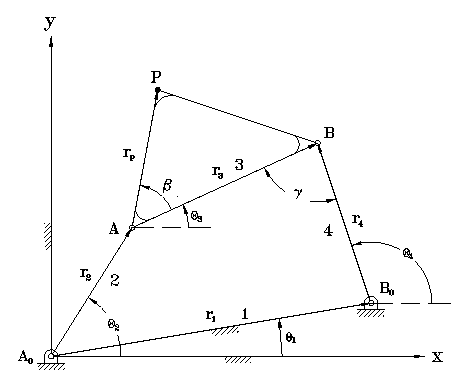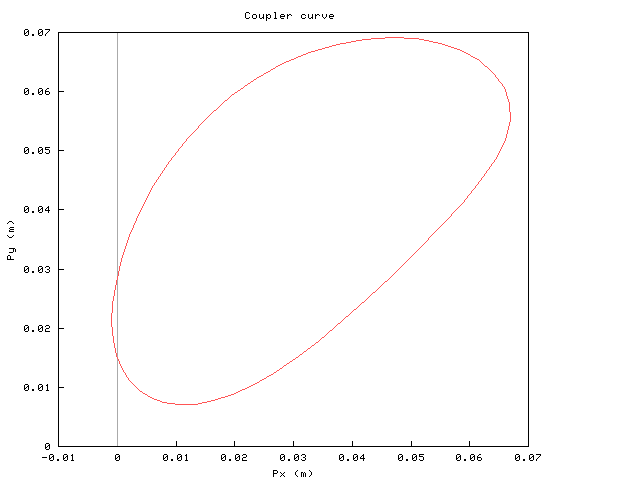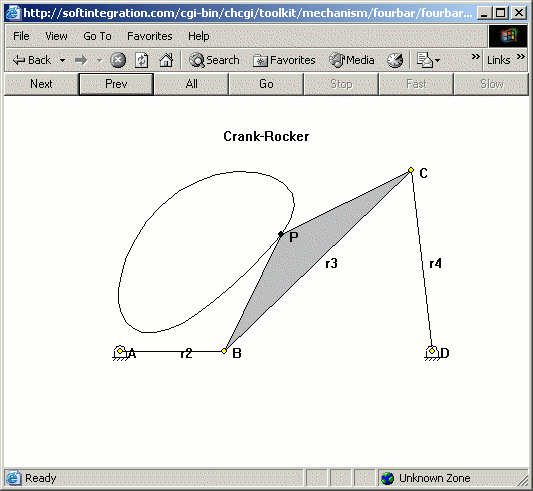SoftIntegration Documentation
 Ch Documents Getting Started Ch-Based Books White Papers ToolKit Documents Ch Mechanism Ch Control System Ch CGI Ch ODBC Library Documents C++ Graphical Library Package Documents Ch NAG Statistics

Getting Started with Ch Mechanism Toolkit

To help you to get familiar with Ch Mechanism Toolkit, a sample program will be used to illustrate basic features and applications of Ch Mechanism Toolkit.

Problem Statement: Link lengths of a fourbar linkage shown below are given as follows: r1 = 12 cm, r2 = 4 cm, r3 = 10 cm, r4 = 7 cm. The phase angle for the ground link is theta_1 = 0, the coupler point P is defined by the distance rp = 5 cm and constant angle beta = 20 degree. Plot a branch of coupler curves for the coupler point and animate the fourbar linkage.This is a crank-rocker four-bar linkage. The code listed below is a Ch program using Ch Mechanism Toolkit for solving this problem. This program is shiped with Ch Mechanism Tolkit. It is located in CHHOME/toolkit/demos/mechanism/chapters/startup/animationcr.ch where CHHOME is the Ch home directory such as C:/ch.

#include <fourbar.h> int main() { /* specify a crank-rocker four-bar linkage */ double r1 = 0.12, r2 = 0.04, r3 = 0.10, r4= 0.07; double theta1 = 0; double rp = 0.05, beta = 20*M_PI/180; int branchnum = 1; class CPlot plot; class CFourbar fourbar; fourbar.setLinks(r1, r2, r3, r4, theta1); fourbar.setCouplerPoint(rp, beta); fourbar.plotCouplerCurve(&plot,branchnum); fourbar.animation(branchnum); return 0; }

The first line of the program